# Glossary of EM.Cube's Materials & Other Physical Object Types

(diff) ← Older revision | Latest revision (diff) | Newer revision → (diff)

## Anisotropic Material

MODULE: EM.Tempo

FUNCTION: Defines an isotropic material object group with four constitutive tensors

TO DEFINE AN ANISOTROPIC MATERIAL GROUP:

1. Right-click on the Anisotropic Materials item in the navigation tree.
2. Select Insert New Isotropic Material... to open up the Isotropic Material dialog.
3. Click the OK button of the dialog to return to the project workspace.

NOTES, SPECIAL CASES OR EXCEPTIONS: EM.Tempo allows you to define a general anisotropic material, whose constitutive parameters, i.e. permittivity (ε), permeability (μ), electrical conductivity (σ) and magnetic conductivity (σm), are all tensorial in nature. Each constitutive parameter in this case is represented by a 3×3 matrix:

A Uniaxial material is a special case of an anisotropic material whose constitutive parameters are all diagonal matrices. Specifying an anisotropic material as Uniaxial in the FDTD Module has a very important computational implication. There are six field update equations for uniaxial materials at each time steps: three for the electric field and three for the magnetic field. In this respect, a uniaxial material is similar to an isotropic dielectric material. On the other hand, a fully anisotropic material with non-zero off-diagonal constitutive matrix elements requires twelve update equations at each time step: three equations for the three components of each of the four vector fields E, D, H and B. As a result, the time loop for fully anisotropic materials takes much longer time than uniaxial materials.

PYTHON COMMAND: None

ANISOTROPIC MATERIAL PARAMETERS

Parameter Name Value Type Units Default Value Notes
Locked mesh density Real Numeric cells/effective wavelength 30 Only if "Lock Mesh" enabled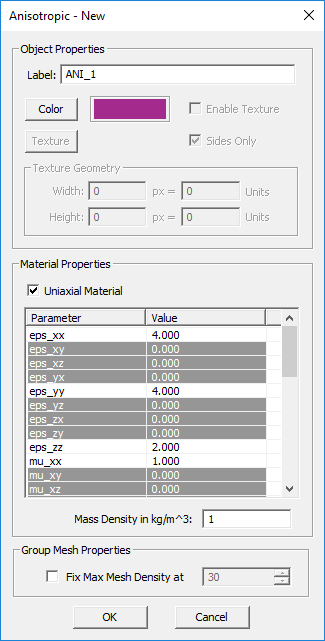The anisotropic material dialog showing the properties of a uniaxial material.

## Dielectric Material

MODULE: EM.Tempo, EM.Ferma, EM.Libera

FUNCTION: Defines a dielectric material object group with specified constitutive parameters

TO DEFINE A DIELECTRIC GROUP:

1. Right-click on the Dielectric Materials or Dielectric Objects item in the navigation tree.
2. Select Insert New Dielectric... to open up the New Dielectric Material dialog.
3. You have two options to choose from: Uniform and Inhomogeneous.
4. If you choose the uniform option, you have to enter numeric values for relative permittivity εr, electric conductivity σ in S/m, relative permittivity μr, magnetic conductivity σm in Ω/m and mass density in kg/m3. The default values are εr = μr = 1, σ = σm = 0, and ρV = 1. You can also enter numeric values for Thermal Conductivity in W/(m.K) and Specific Heat Capacity in J/(kg.K). These parameters are used for thermal simulation in EM.Ferma.
5. If you choose the inhomogeneous option, you have to enter mathematical or Python expressions in the global (x,y,z) coordinates for all the constituent parameters.
6. Change the color of the material group if necessary.
7. Click the OK button of the dialog to return to the project workspace.

NOTES, SPECIAL CASES OR EXCEPTIONS: The inhomogeneous option is available only in EM.Tempo and EM.Ferma. Although in this mode you may enter numeric values as valid mathematical or Python expressions, beware of the fact that the Python expressions are evaluated at every single mesh point. This may take a very long processing time for large meshes.

PYTHON COMMAND: dielectric_group(label,eps,sigma,mu,sigma_m[,rr,gg,bb])

DIELECTRIC PARAMETERS

Parameter Name Value Type Units Default Value Notes
eps (εr) Real Numeric - 1.0 relative permittivity
sigma (σ) Real Numeric S/m 0.0 electric conductivity
mu (μr) Real Numeric - 1.0 relative permeability
sigma_m (σm) Real Numeric Ohm/m 0.0 magnetic conductivity
loss_tangent Real Numeric - 0.0 dielectric loss tangent
mass_dens (ρV) Real Numeric kg/m3 1.0 volume mass density
thermal_cond Real Numeric W/(m.K) 0.0 thermal conductivity
spec_cap Real Numeric J/(kg.K) 0.0 specific heat capacity
Locked mesh density Real Numeric cells/effective wavelength 30 Only if "Lock Mesh" enabled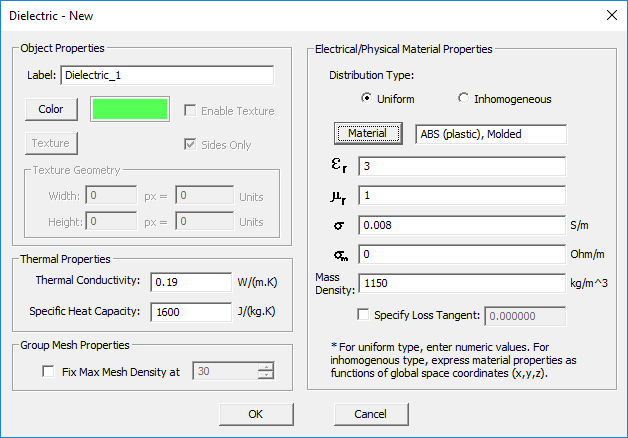The dielectric material dialog showing a uniform dielectric material.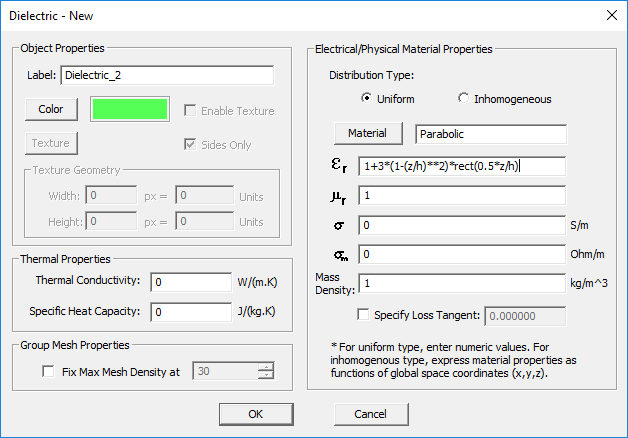The dielectric material dialog showing an inhomogeneous dielectric material with a parabolic permittivity profile. In the expression, h is a project variable and rect(z) is the pulse function.

## Dispersive Material

MODULE: EM.Tempo

FUNCTION: Defines a dispersive material object group with specified poles

TO DEFINE A DISPERSIVE MATERIAL GROUP:

1. Right-click on the Dispersive Materials item in the navigation tree.
2. Select Insert New Debye Material... or Insert New Drude Material... or Insert New Lorentz Material... or Insert New Generalized Metamaterial... or to open up one of the four Dispersive Material dialogs.
3. You have to define each pole of the dispersive material individually. You can add as many poles as you want using the Add Pole button of the dialog.
4. Click the OK button of the dialog to return to the project workspace.

NOTES, SPECIAL CASES OR EXCEPTIONS: EM.Tempo currently offers four types of dispersive material:

1. Debye Material
2. Drude Material (Unmagnetized Plasma)
3. Lorentz Material
4. Generalized Metamaterial

The FDTD simulation engine uses the Auxiliary Differential Equation (ADE) method to model dispersive materials. EM.Cube allows you to define an arbitrary number of poles for each of the above dispersive material types. Keep in mind that all the objects belonging to the same dispersive material group have the same dispersion properties.

The complex permittivity of a Debye material with N poles is given by:

$\varepsilon (\omega) = \varepsilon_\infty + \sum_{p=1}^N \dfrac{\Delta \varepsilon_p}{1 + j\omega \tau_p}, \quad \Delta \varepsilon_p = \varepsilon_{sp} - \varepsilon_\infty$

where $\varepsilon_{\infty}$ is the value of the permittivity at infinite frequency, $\tau_p$ is the relaxation time corresponding to the pth pole having the unit of seconds, and $\varepsilon_{sp}$ is the value of the static permittivity (at DC) corresponding to the pth pole. $\Delta \varepsilon_p = \varepsilon_{sp} - \varepsilon_{\infty}$ represents the change in permittivity due to the pth pole.

Unmagnetized plasmas are typically modeled as Drude materials. The complex permittivity of a Drude material with N poles is given by:

$\varepsilon(\omega) = \varepsilon_{\infty} - \sum_{p=1}^N \dfrac{{\omega_p}^2}{\omega^2 - j\omega \nu_p}$

where $\omega_p$ and $\nu_p$ are the angular plasma frequency and angular collision frequency corresponding to the pth pole, respectively, and both are expressed in rad/s. For an unmagnetized plasma, $\varepsilon_{\infty} = 1$.

The complex permittivity of a Lorentz material with N poles is given by:

$\varepsilon(\omega) = \varepsilon_{\infty} - \sum_{p=1}^N \dfrac{\Delta \varepsilon_p {\omega_p}^2}{\omega^2 - 2j\omega \delta_p - {\omega_p}^2}, \quad \Delta \varepsilon_p = \varepsilon_{sp} - \varepsilon_{\infty}$

where $\omega _p$ and $\delta_p$ are the angular resonant frequency and angular damping frequency corresponding to the pth pole, respectively, and both are expressed in rad/s. Similar to a Debye material, $\Delta \varepsilon_p = \varepsilon_{sp} - \varepsilon_{\infty}$ represents the change in permittivity due to the pth pole.

The Debye, Drude and Lorentz dispersive materials are all isotropic dielectric materials having a scalar relative permittivity that varies with the frequency. A generalized metamaterial in EM.Tempo is defined as a uniaxial dispersive material that has three relative permittivities εr,xx, εr,yy, εr,zz and three relative permeabilities μr,xx, μr,yy, and μr,zz. These six quantities can independently be functions of the frequency in general or non-dispersive. Moreover, you can define poles of different kind for each constitutive parameter. The pole type can be Debye, Drude or Lorentz.

PYTHON COMMAND: None

DISPERSIVE MATERIAL PARAMETERS

Parameter Name Value Type Units Default Value Notes
Locked mesh density Real Numeric cells/effective wavelength 30 Only if "Lock Mesh" enabled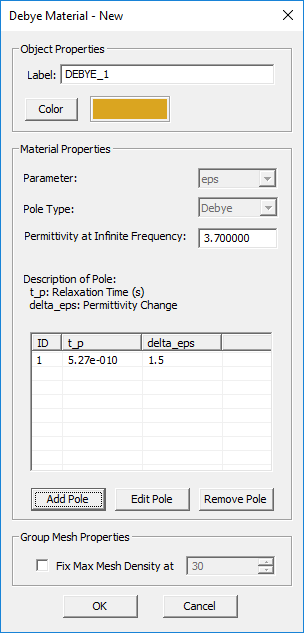The Debye dispersive material dialog.Defining the parameters of a Debye-Type pole in the "Add Pole" dialog.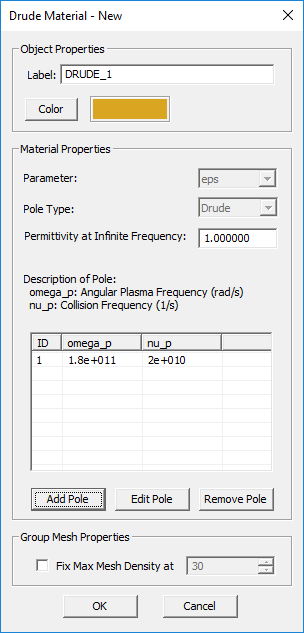The Drude dispersive material dialog.Defining the parameters of a Drude-Type pole in the "Add Pole" dialog.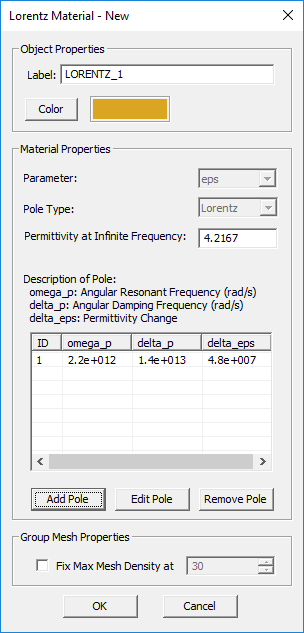The Lorentz dispersive material dialog.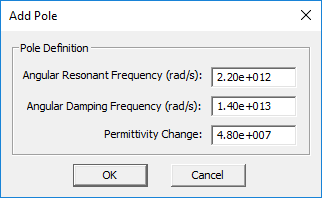Defining the parameters of a Lorentz-Type pole in the "Add Pole" dialog.Defining the parameters of a Drude-Type pole for εr,xx in the "Add Pole" dialog.

## Ferrite Material

MODULE: EM.Tempo

FUNCTION: Defines a ferrite material

TO DEFINE A FERRITE MATERIAL GROUP:

1. Right-click on the Gyrotropic Materials item in the navigation tree.
2. Select Insert New Ferrite Material... to open up the New Ferrite dialog.
3. Enter values for the DC Bias Magnetic Field and Saturation Magnetization.
4. If necessary, change the default values of attenuation factor, permittivity and electric conductivity.
5. Change the color of the material group if desired.
6. Clicking the Plot button generates plots of the components of the permeability tensor over the frequency range of the project.
7. Click the OK button of the dialog to return to the project workspace.

PYTHON COMMAND: None

VOXEL DATABASE PARAMETERS

Parameter Name Value Type Units Default Value Notes
DC Bias Magnetic Field Real Numeric A/m 100000 Assumed to be Z-directed
Saturation Magnetization Real Numeric A/m 50000 Assumed to be Z-directed
Attenuation Factor Real Numeric - 0 -
eps (εr) Real Numeric - 1.0 relative permittivity
sigma (σ) Real Numeric S/m 0.0 electric conductivity
Locked mesh density Real Numeric cells/effective wavelength 30 Only if "Lock Mesh" enabled

## Gyrotropic Material

MODULE: EM.Tempo

FUNCTION: Defines a gyrotropic material (either a ferrite or a magnetoplasma)

TO DEFINE A GYROTROPIC MATERIAL GROUP:

1. Right-click on the Gyrotropic Materials item in the navigation tree.
2. Select either Insert New Ferrite Material... or Insert New Magnetoplasma... to open up the New Ferrite dialog or New Magneoplasma dialog, respectively.
3. For a ferrite material, follow the procedure at Ferrite Material.
4. For a magnetoplasma material, follow the procedure at Magnetoplasma Material.
5. Change the color of the material group if desired.
6. Click the OK button of the dialog to close it.

NOTES, SPECIAL CASES OR EXCEPTIONS: In the case of a magnetoplasma, the user has two options, either to specify the DC magnetic field or the angular cyclotron frequency. Once either of the two parameter is specified, the value of the other parameter is computed and displayed.

PYTHON COMMAND: None

FERRITE MATERIAL PARAMETERS

Parameter Name Value Type Units Default Value Notes
DC bias magnetic field Real Numeric A/m 105 assumed to be Z-directed
saturation magnetization Real Numeric A/m 5×104 assumed to be Z-directed
attenuation factor Real Numeric - 0 must be between 0 and 1
relative permittivity Real Numeric - 1 -
electric conductivity Real Numeric S/m 0 -
Locked mesh density Real Numeric cells/effective wavelength 30 Only if "Lock Mesh" enabled

MAGNETOPLASMA MATERIAL PARAMETERS

Parameter Name Value Type Units Default Value Notes
angular plasma frequency Real Numeric rad/s 3.14×107 -
collision frequency Real Numeric 1/s 105 -
DC bias magnetic field Real Numeric A/m 105 assumed to be Z-directed
angular cyclotron frequency Real Numeric rad/s 8837887.024 must be specified if the DC magnetic field check box is unchecked
Locked mesh density Real Numeric cells/effective wavelength 30 Only if "Lock Mesh" enabled

## Magnetoplasma Material

MODULE: EM.Tempo

FUNCTION: Defines a magnetoplasma material

TO DEFINE A FERRITE MATERIAL GROUP:

1. Right-click on the Gyrotropic Materials item in the navigation tree.
2. Select Insert New Magnetoplasma... to open up the New Magnetoplasma dialog.
3. Enter values for the Angular Plasma Frequency and Collision Frequency.
4. The box labeled "Specify the Z-directed DC Magnetic Field" is checked by default. In that case, you need to enter a value for the DC magnetic field.
5. If you uncheck the magnetic field box, then you need to enter a value for Angular Cyclotron Frequency.
6. Change the color of the material group if desired.
7. Clicking the Plot button generates plots of the components of the permittivity tensor over the frequency range of the project.
8. Click the OK button of the dialog to return to the project workspace.

PYTHON COMMAND: None

VOXEL DATABASE PARAMETERS

Parameter Name Value Type Units Default Value Notes
Angular Plasma Frequency Real Numeric rad/s 31400000 -
Collision Frequency Real Numeric 1/s 100000 -
DC Magnetic Field Real Numeric A/m 40 Assumed to be Z-directed
Angular Cyclotron Frequency Real Numeric rad/s 8837887.024 Disabled when specifying the DC magnetic field
Locked mesh density Real Numeric cells/effective wavelength 30 Only if "Lock Mesh" enabled

## Penetrable Surface

MODULE: EM.Terrano

FUNCTION: Defines a penetrable surface (thin wall) block group of a specified thickness

TO DEFINE AN PENETRABLE SURFACE GROUP:

1. Right-click on the Penetrable Surfaces item in the navigation tree.
2. Select Insert New Block... to open up the Penetrable Surface dialog.
3. In the material table, the default setting shows Brick with a relative permittivity of εr = 4.44, an electric conductivity of σ = 0.001S/m and a thickness of 0.5 project units.
4. You can change the default material composition by selecting and highlighting it in the table and clicking the Add/Edit button of the dialog.
5. In the Material Layer Properties dialog, either enter new values for εr and σ or click the Material button of this dialog to open EM.Cube's Materials List and select one of its entries. You can also change the Wall Thickness from this dialog.
6. You can add several layers to your penetrable wall composition. An incident ray first penetrates the topmost layer and finally exits the bottommost layer.
7. After you complete the definition of all layers, click the OK button of the dialog to return to the project workspace.

PYTHON COMMAND: penetrable_surface_group(label,epsilon,sigma,thickness[,rr,gg,bb])

PENETRABLE SURFACE PARAMETERS

Parameter Name Value Type Units Default Value Notes
epsilon Real Numeric - 4.44 relative permittivity
sigma Real Numeric S/m 1e-3 electric conductivity
thickness Real Numeric project units 0.5 finite thickness of thin walls
Locked mesh density Real Numeric cells/effective wavelength 30 Only if "Lock Mesh" enabled

## Penetrable Volume

MODULE: EM.Terrano

FUNCTION: Defines a penetrable volume block group

TO DEFINE AN PENETRABLE VOLUME GROUP:

1. Right-click on the Penetrable Volumes item in the navigation tree.
2. Select Insert New Block... to open up the Penetrable Volume dialog.
3. From the Interface drop-down dialog, choose one of the four options: Standard Material, Rain, Fog, Vegetation.
4. The default interface type is the standard material Brick with a relative permittivity of εr = 4.44, an electric conductivity of σ = 0.001S/m.
5. You can change the default material composition by entering new values for εr and σ. Or click the Material button of this dialog to open EM.Cube's Materials List and select one of its entries.
6. If you choose the Rain option, you have to enter a value for Rain Rate in mm/hr.
7. If you choose the Fog option, you have to enter a value for Fog Density in g/m^3.
8. If you choose the Vegetation option, Weissberger's model will be used and there is no parameter to set.
9. After you complete the definition of all layers, click the OK button of the dialog to return to the project workspace.

NOTES, SPECIAL CASES OR EXCEPTIONS: Inside a penetrable volumes, the rays travel through a non-air medium. As a result, they experience different phase velocities and possibly additional losses. Therefore, the attenuation and propagation constants of the material medium are used for wave computations:

$\gamma = \alpha + j\beta = \alpha + j\frac{\omega}{c}\sqrt{\epsilon_r}$

where α and β are the attenuation and propagation constants in Neper/m and radian/m, respectively, ω = 2*πf, εr is the relative permittivity of the medium and f the operational frequency.

There are four types of penetrable volumes:

• Standard material
• Rain
• Fog
• Vegetation

Standard material blocks reflect, transmit and diffract impinging rays. The ray reflection and edge diffraction mechanisms are identical to the cases of impenetrable or penetrable surfaces with the same material properties. The transmitted rays, however, travel in the material medium until they exit the other side of the penetrable volume object. This is different than the case of penetrable surfaces, where the transmitter ray is assumed to travel the very short thickness of a wall before entering the free space again, and the specular points of the entering and exiting rays are taken to be the same.

Of the above list, rain, fog and vegetation media only attenuate the incoming ray and do not generate reflected or diffracted rays. A rain medium is characterized by the Rain Rate expressed in mm/hr, and a fog medium is characterized by the Fog Density expressed in gr/m3. EM.Terrano uses Weissberger's model for the calculation of attenuation due to propagation in a vegetation medium. This model is only applicable when there is foliage obstruction (blockage by dense, dry and leafy trees) in a line-of-sight link. Weissberger's model is valid in the frequency range from 230MHz to 95GHz and provides accurate results for foliage depths up to 400m. It expressed the propagation loss as

$L = \left\{ \begin{array}{lr} 0.45 f^{0.284}d & d\lt14\text{m} \\ 1.33 f^{0.284}d^{0.588} & 14\text{m} \le d \le 400\text{m} \end{array} \right.$

where L is the total loss in dB, d is the depth of the foliage and f is the operational frequency in GHz.

PYTHON COMMAND: penetrable_volume_group(label,epsilon,sigma[,rr,gg,bb])

PENETRABLE VOLUME PARAMETERS

Parameter Name Value Type Units Default Value Notes
eps Real Numeric - 4.44 relative permittivity
sigma Real Numeric S/m 1e-3 electric conductivity
Locked mesh density Real Numeric cells/effective wavelength 30 Only if "Lock Mesh" enabled

## Perfect Electric Conductor (PEC)

FUNCTION: Defines a perfect electric conductor object group

TO DEFINE A PEC GROUP:

1. Right-click on the PEC Objects or PEC Traces item in the navigation tree.
2. Select Insert New PEC... to open up the New PEC Dialog.
3. The only properties of a PEC group you can modify is its color and texture.
4. Click the OK button of the dialog to return to the project workspace.

PYTHON COMMAND: pec_group(label)

PEC PARAMETERS

Parameter Name Value Type Units Default Value Notes
Locked mesh density Real Numeric cells/effective wavelength 30 Only if "Lock Mesh" enabled

## Perfect Magnetic Conductor (PMC)

MODULE: EM.Tempo, EM.Illumina

FUNCTION: Defines a perfect magnetic conductor object group

TO DEFINE A PMC GROUP:

1. Right-click on the PMC Planes or PMC Surfaces item in the navigation tree.
2. Select Insert New PMC... to open up the New PMC dialog.
3. The only properties of a PMC group you can modify is its color and texture.
4. Click the OK button of the dialog to return to the project workspace.

PYTHON COMMAND: pmc_group(label)

PMC PARAMETERS

Parameter Name Value Type Units Default Value Notes
Locked mesh density Real Numeric cells/effective wavelength 30 Only if "Lock Mesh" enabled

## Permanent Magnet

MODULE: EM.Ferma

FUNCTION: Defines a permanent magnet group with a specified magnetization vector

TO DEFINE A PERMANENT MAGNET GROUP:

1. Right-click on the Permanent Magnets item in the navigation tree.
2. Select Insert New Permanent Magnet... to open up the Permanent Magnet Source dialog.
3. Enter a value for relative permeability μr, which has a default value of 1.
4. You have two options for Magnetization to choose from: Uniform and Inhomogeneous.
5. If you choose the uniform option, you have to enter three numeric values for the three components of the magnetization vector Mx, My and Mz in A/m.
6. If you choose the inhomogeneous option, you have to enter three expressions for the three components of the magnetization vector Mx, My and Mz in the global (x,y,z) coordinates.
7. Click the OK button of the dialog to return to the project workspace.

NOTES, SPECIAL CASES OR EXCEPTIONS: A permanent magnet is typically a ferromagnetic material with a fixed intrinsic magnetization vector. As a result, it can be used as a source in an magnetostatic problem. When a permeable material has a permanent magnetization, the following relationship holds:

$\mathbf{B(r)} = {\mu} (\mathbf{H(r)} + \mathbf{M(r)} )$

where M(r) is the magnetization vector. In SI units system, the magnetic field H and magnetization M both have the same units of A/m.

It can be shown that for magnetostatic analysis, the effect of the permanent magnetization can be modeled as an equivalent volume current source:

$\mathbf{J_{eq}(r)} = \nabla \times \mathbf{M(r)}$

If the magnetization vector is uniform and constant inside the volume, then its curl is zero everywhere inside the volume except on its boundary surface. In this case, the permanent magnetic can be effectively modeled by an equivalent surface current density on the surface of the permanent magnetic object:

$\mathbf{J_{s,eq}(r)} = \mathbf{M(r)} \times \hat{\mathbf{n}}$

where $\hat{\mathbf{n}}$ is the unit outward normal vector at the surface of the permanent magnet object. Note that the volume of the permanent magnet still acts as a permeable material in the magnetostatic analysis.

PYTHON COMMAND: magnet_group(label,mu,Mx,My,Mz)

PERMANENT MAGNET PARAMETERS

Parameter Name Value Type Units Default Value Notes
mu (μr) Real Numeric - 1.0 relative permeability
Mx Real Numeric A/m 0.0 X-component of magnetization vector
My Real Numeric A/m 0.0 Y-component of magnetization vector
Mz Real Numeric A/m 1.0 Z-component of magnetization vector

## Terrain Surface

MODULE: EM.Terrano

FUNCTION: Defines a terrain surface block group

TO DEFINE A TERRAIN SURFACE GROUP:

1. Right-click on the Terrain Surfaces item in the navigation tree.
2. Select Insert New Terrain... to open up the Terrain Surface dialog.
3. In the material table, the default setting shows Rock with a relative permittivity of εr = 5 and an electric conductivity of σ = 0.001S/m.
4. You can change the default material composition by selecting and highlighting it in the table and clicking the Add/Edit button of the dialog.
5. In the Material Layer Properties dialog, either enter new values for εr and σ or click the Material button of this dialog to open EM.Cube's Materials List and select one of its entries.
6. You can add several layers to your terrain surface composition. In that case, you need to define a thickness for each layer. The bottommost layer always has an infinite thickness representing an unbounded half-space medium as seen by an incident ray.
7. After you complete the definition of all layers, click the OK button of the dialog to return to the project workspace.

PYTHON COMMAND: terrain_group(label,epsilon,sigma[,rr,gg,bb])

TERRAIN SURFACE PARAMETERS

Parameter Name Value Type Units Default Value Notes
epsilon Real Numeric - 5 relative permittivity
sigma Real Numeric S/m 5e-3 electric conductivity
Locked mesh density Real Numeric cells/effective wavelength 30 Only if "Lock Mesh" enabled

## Virtual Object Group

MODULE: EM.Terrano

FUNCTION: Defines a virtual object group that will be invisible to the simulation engine

TO DEFINE A VIRTUAL OBJECT GROUP:

1. Right-click on the Virtual Objects item in the navigation tree.
2. Select Insert a Virtual Material... to open up the Virtual Material dialog.
3. Virtual materials have only one property, which is their color. You can the change from this dialog.
4. Click the OK button of the dialog to return to the project workspace.

PYTHON COMMAND: virtual_group(label)

VIRTUAL OBJECT PARAMETERS: None